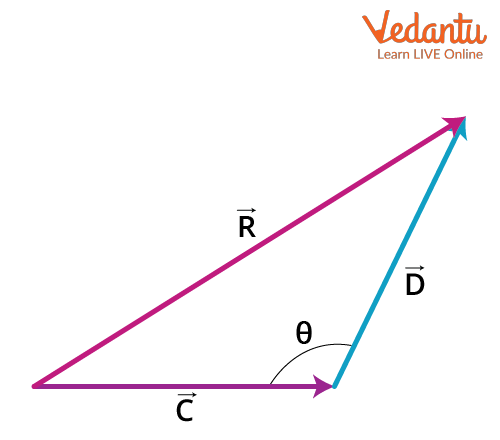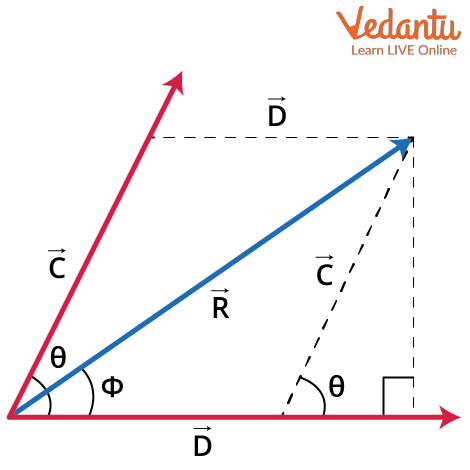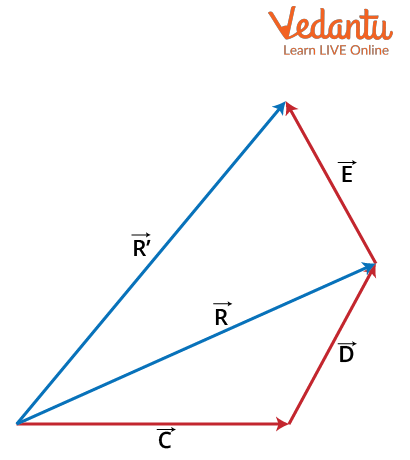Courses
Courses for Kids
Free study material
Free LIVE classes
More

# Addition of Three Vectors - Laws and Solved Examples for JEELast updated date: 21st Mar 2023
Total views: 74.7k
Views today: 0.53k

As we know,  a vector is defined as a physical quantity that has both direction and magnitude. Any vector can be represented in the XYZ plane using its components. If two vectors are defined by giving their direction components on the XYZ plane and if they are being added, we can just add the X components, Y components and Z components of two vectors, respectively. When the vectors are defined by their magnitude, we have two different laws of vector addition through which the vectors can be added.

These are the analytical methods used for the vector addition and they are the Triangle Law and the Parallelogram Law of vector addition. Let us have an overview of how the Triangle Law and the Parallelogram Law are carried out in the vector addition.

### Triangle Law

The Triangle Law of vector addition states that if two vectors are drawn as the two adjacent sides of the triangle both in magnitude and direction, then the third side of the triangle is the resultant of these two vectors after addition both in magnitude and direction. Let's take two vectors, namely C and D and draw them as the two orderly sides of the triangle as shown in the below figure.In the above figure, R is the resultant vector after adding two vectors C and D.

The magnitude of the resultant vector by the Triangle Law is given by the equation as follows, $R=\sqrt{c^{2}+d^{2}+2 c d \cos \theta}$

Here, c and d are the magnitudes of two vectors C and D respectively. θ is the angle between two vectors C and D. Let's have a look at the parallelogram law of vector addition with also some vector addition examples.

### Parallelogram Law

The Parallelogram Law of vector addition states that if two vectors, both in magnitude and direction, are represented as the two adjacent sides of the parallelogram, then the diagonal passing through these two vectors is at rest at the resultant of the vectors after addition. Let us have a demonstration of the Parallelogram Law with the help of a diagram.In the above figure, R represents the resultant vector after adding two vectors C and D which is the diagonal of the parallelogram. Here, $\theta$ is the angle between two vectors C and D whereas ɸ is the angle between the resultant vector and the vector D. The magnitude of the resultant is given by the equation,

$R=\sqrt{c^{2}+d^{2}+2 c d \cos \theta}$

If we want to know the angle between the resultant vector  and the vector D, the relation between $\phi$ and $\theta$ is given by,

$\tan \phi=\dfrac{c \sin \theta}{d+c \cos \theta}$

These are the vector addition formulae used with the law of addition of vectors.

Let us assume that there are three vectors C, D and E, which are to be added through the law of vector addition formulae. For the addition of the three vectors, we can make the vector addition for the first two vectors C and D, where we obtain the resultant R. Then add this result to the third vector, E to obtain the final resultant of the addition of three vectors, i.e., R’.This is how the three vectors are added using the laws of vector addition and this process is also similar for the subtraction of vectors. The following provides example problems to understand how the three vectors are added.

Example 1: Given that the magnitude of vectors, |C| = 4units, |D| = 5 units and |E| = 7 units respectively. Find the magnitude of the resultant vector after the addition of three vectors and given the angle between C and D is 90 degrees and the angle between R and E is 30 degrees.

Solution:

Given that

|C| = 4, |D| = 5 and |E| = 7

$\theta$  = angle between C and D = 90o

Let us find the magnitude of the resultant vector,  C and D.

$|R|=\sqrt{c^{2}+d^{2}+2 c d \cos \theta}=\sqrt{4^{2}+5^{2}+2(5)(4) \cos 90}=\sqrt{41} \text { units }$

Hence, we have got the magnitude of the resultant vector between C and D.

Let us now find the magnitude of the resultant vector, R’ which is the addition of two vectors, R and E. Let us assume the angle between the vectors, R and E is $\alpha$ = 30

I.e.,

$\left|R^{\prime}\right|=\sqrt{R^{2}+E^{2}+2 R E \cos \alpha}$

Therefore, by putting the values of R, E and $\alpha$ in the above equation, we obtain

\begin{align} &\left|R^{\prime}\right|=\sqrt{(\sqrt{41})^{2}+(7)^{2}+2(7) \sqrt{41} \cos 60} \\ &=\sqrt{41+49+7 \sqrt{41}} \\ &=11.611 \text { units } \end{align}

This is the required value of the resultant vector after adding the three vectors, C, D and E.

Example 2: Three vectors are given by their X, Y and Z components as follows.

C = 2i + 4j + 7k

D = i + 3j + 8k

E = -4i + 2j - 5k

Find the magnitude of the resultant vector after adding these three vectors.

Solution:

We can add three vectors by their respective components and write the resultant vector as, R = Rxi + Ryj + Rz

Where, Rx = (Cx + Dx + Ex) = [2 + 1 + (-4)] = -1

Ry = (Cy + Dy + Ey) = [4 + 3 + 2] = 9

Rz = (Cz + Dz + Ez) = [7 + 8 + (-5)] = 10

Therefore, the resultant vector, R = -i + 9j + 10k

The magnitude of the resultant vector, R is given by the following equation,

$|R|=\sqrt{R_{x}^{2}+R_{y}^{2}+R_{z}^{2}}=\sqrt{(-1)^{2}+(9)^{2}+(10)^{2}}=\sqrt{182}=13.49 \text { units }$

The required value of the magnitude of the resultant of three vectors is 13.49units.

## Conclusion

Generally, the vectors are added following two methods which are namely analytical and graphical methods. These methods can be used for both addition and subtraction of vectors. We know that the addition of two vectors is done with the help of the Triangle Law and Parallelogram Law of addition of vectors. We learnt how to calculate the addition of vectors using the vector addition formula with the help of vector addition examples in this article.

## FAQs on Addition of Three Vectors - Laws and Solved Examples for JEE

1. What are the properties of vector addition?

There are some properties of vector addition which are followed by every vector that is being added. Let us assume the two vectors which are being added are C and D. The first property is the commutative property, which states that C + D = D + A. The second property is the associative property which consists of three vectors, C, D and E and states that C + (D+E) = (C+D) + E. The third property is the inverse property which expresses that if a positive vector is added to its negative form then the result obtained is zero. I.e., C + (-C) = 0.

2. What is the weightage of this topic in JEE?

The topic of vectors, the addition of vectors and the subtraction of vectors is very important for the JEE examination. The Triangle Law and the Parallelogram Law of vector addition and subtraction are very essential and basics for the in-depth concepts of vectors. A maximum of 1 to 2 questions will be asked in the JEE with a weightage of 6.6 %. Learning and understanding the law of addition of vectors is very important which we have learnt in this article.